# Duistermaat & Kolk .... Vol II .... Proof of Proposition 6.1.2

• I
• Math Amateur

#### Math Amateur

Gold Member
MHB
TL;DR Summary
I am reading Multidimensional Real Analysis II (Integration) by J.J. Duistermaat and J.A.C. Kolk ... and am focused on Chapter 6: Integration ...

I need some help with aspects of the proof of Proposition 6.1.2 ...
I am reading Multidimensional Real Analysis II (Integration) by J.J. Duistermaat and J.A.C. Kolk ... and am focused on Chapter 6: Integration ...

I need some help with the proof of Proposition 6.1.2 ...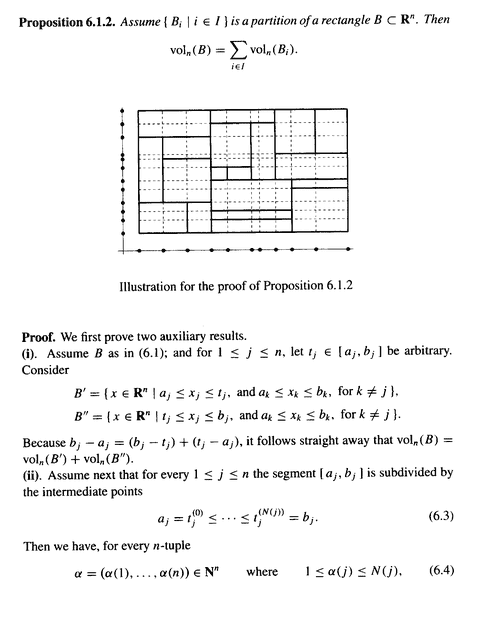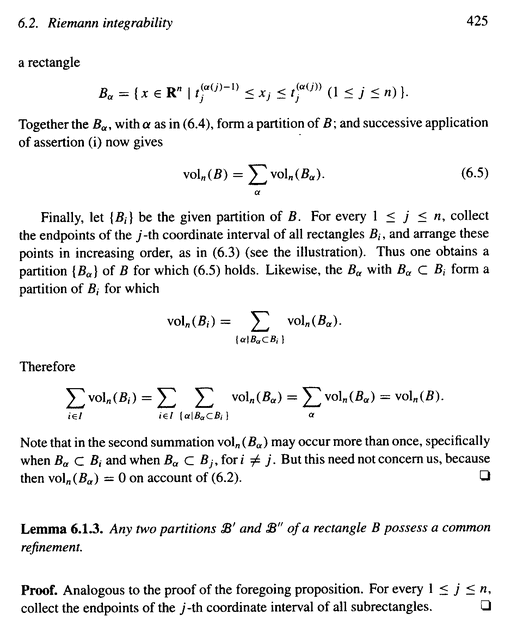Definitions and text preliminary to the Proposition reads as follows: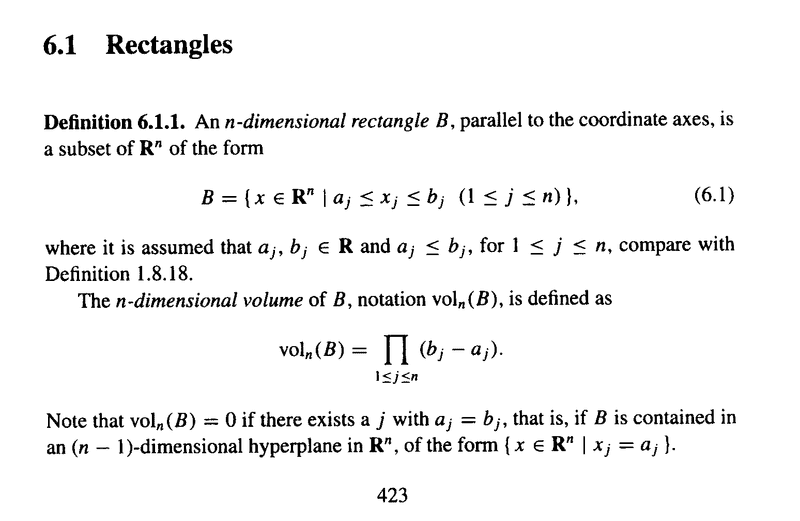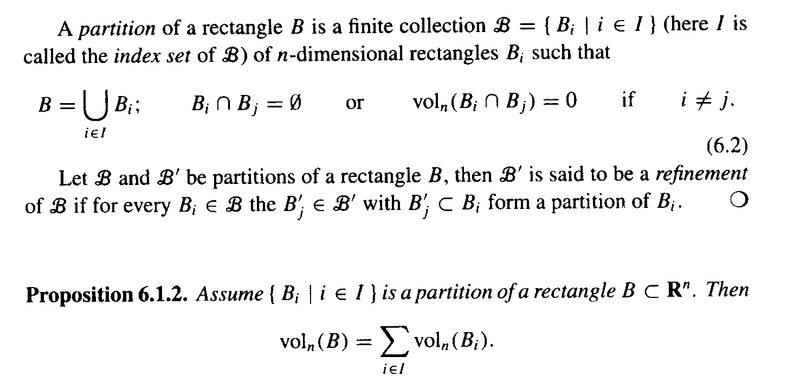I am trying to write a detailed proof of the fact or assertion that

$\text{vol}_n (B) = \text{vol}_n (B') + \text{vol}_n (B'')$ ... ... ... ... (*)

given their definitions as sets ... but have so far been able to formulate a proof ...

I hope someone can help ...

I am especially interested in tying or connecting the proof of (*) to the definitions of B' and B" as sets.

To explicitly indicate my concerns I am presenting an example from $$\mathbb{R^2}$$

Let $B' = \{ ( x_1, x_2 ) \in \mathbb{R^2} \; \; | \; \; a_1 \leq x_1 \leq t_1 \text{ and } a_2 \leq x_2 \leq b_2 \; \; ; \; \; \text{ and } a_2 \leq x_2 \leq t_2 \text{ and } a_1 \leq x_1 \leq b_1 \}$

Thus B' is made up of two rectangles, viz.

$B'_1 = \{ ( x_1, x_2 ) \in \mathbb{R^2} \; \; | \; \; a_1 \leq x_1 \leq t_1 \text{ and } a_2 \leq x_2 \leq b_2 \}$

and

$B'_2 = \{ ( x_1, x_2 ) \in \mathbb{R^2} \; \; | \; \; a_2 \leq x_2 \leq t_2 \text{ and } a_1 \leq x_1 \leq b_1 \}$

These two rectangles are depicted in Figures 1 and 2 below ...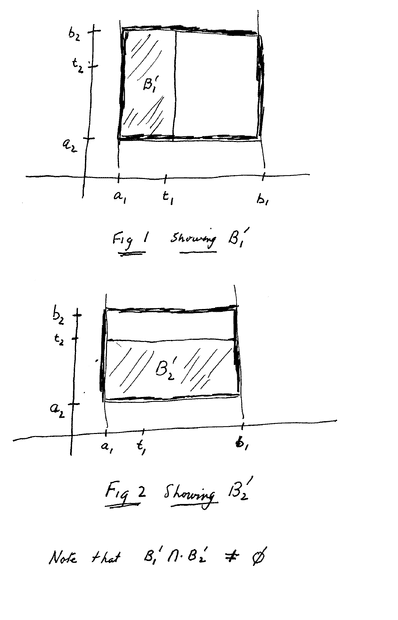Now ... let $B" = \{ ( x_1, x_2 ) \in \mathbb{R^2} \; \; | \; \; t_1 \leq x_1 \leq b_1 \text{ and } a_2 \leq x_2 \leq b_2 \; \; ; \; \; \text{ and } t_2 \leq x_2 \leq b_2 \text{ and } a_1 \leq x_1 \leq b_1 \}$

Thus $B"$ is made up of two rectangles, viz.
$B"_1 = \{ ( x_1, x_2 ) \in \mathbb{R^2} \; \; | \; \; t_1 \leq x_1 \leq b_1 \text{ and } a_2 \leq x_2 \leq b_2 \}$

and

$B"_2 = \{ ( x_1, x_2 ) \in \mathbb{R^2} \; \; | \; \; t_2 \leq x_2 \leq b_2 \text{ and } a_1 \leq x_1 \leq b_1 \}$

The above two rectangles $B"_1$ and $B"_2$ are depicted in Figures 3 and 4 below ...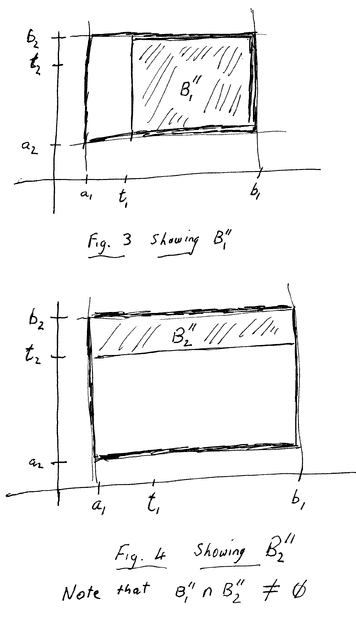Now ... my problem is this: I do not see how

$\text vol_n (B) =\text vol_n (B') + \text vol_n (B'')$ ... ... ... ... (*)

... that is I do not see how the volumes of $B'$ and $B"$ which together comprise the overlapping rectangles $B'_1 , B'_2 , B"_1 \text{ and } B"_2$ satisfy (*) ...

Can someone explain how (*) can be true given my analysis above ... I must be making some errors ...

NOTE: I know that (6.2) in the text in defining the conditions for a partition of a rectangle Duistermaat and Kolk write

$B_i \cap B_j = \emptyset$ or $\text vol_n ( B_i \cap B_j ) = 0$ if $i \neq j$

... but this is prescriptively defining a partition of a rectangle $B$... it does not, in my opinion, mean that we can overlook (put to zero) the overlaps or intersections in the rectangles $B'_1 , B'_2, B"_1, \text{ and } B"_2$ ...

Either way I cannot see how $\text vol_n (B) =\text vol_n (B') + \text vol_n (B'')$ ... ... ... ... (*)

arises from the union of the rectangles

$B'_1 , B'_2, B"_1, \text{ and } B"_2$ ...

Hope someone can explain and clarify this aspect of the proof of Proposition 6.1.2 ...

Peter

#### Attachments

Last edited:

Looks like you started using one dollar instead of two.

Thanks PeroK ... yes, you are right ... thanks ... will correct

Math Amateur

Just to write down the definitions, you have
##\text{vol}(B)=(a_2-a_1)(b_2-b_1)##
And
##\text{vol}(B_1')=(t_1-a_1)(b_2-b_1)##, ##\text{vol}(B_1'')=(a_2-t_1)(b_2-b_1)##

And you aren't sure how to prove ##\text{vol}(B)=\text{vol}(B_1')+\text{vol}(B_1'')##?

Thanks PeroK ... yes, you are right ... thanks ... will correct

Math Amateur
Just to write down the definitions, you have
##\text{vol}(B)=(a_2-a_1)(b_2-b_1)##
And
##\text{vol}(B_1')=(t_1-a_1)(b_2-b_1)##, ##\text{vol}(B_1'')=(a_2-t_1)(b_2-b_1)##

And you aren't sure how to prove ##\text{vol}(B)=\text{vol}(B_1')+\text{vol}(B_1'')##?
Yes ... can see where you are going with this ... and i will check it ...

BUT

I am puzzled by my two dimensional example ... the volumes of the rectangles that come out of B' and B" (see Figures 1 to 4 ...) should add to the volume of B ... but it does not appear to be the case ... but why ..

... in other words ... if you work from the basic set definitions of B, B' and B" you should also come up with the sum of the volumes of B' and B" being equal to the volume of B ... but my analysis doesn't seem to show this ..

... can you clarify what is going on ...

Peter

It's just a little bit of algebra. Add the two volumes together, and factor the ##b_2-b_1##.

What makes you say they don't add up?

Well … my very basic example should add up … it proceeds from the basic set theory definitions of B’ and B” … yet the volumes you get for B’ and B” do not seem to add up …so something is wrong because your proof shows it does add up to Volume of B …

so I’m hoping you can explain to me how my 2 dimensional case shows (*) is true in 2 dimensions… but in my example it does not appear that the volumes of B’ and B” do not add up to the volume of B … se my 2-dimensional example … what am I doing wrong …

it is not so much a question of a proof … it is more a question of understanding and also understanding the approach of Duistermaat and Kolk …

can you help me to understand why my two dimensional example seems to go wrong in calculating volumes …

Math Amateur

Well … my very basic example should add up … it proceeds from the basic set theory definitions of B’ and B” … yet the volumes you get for B’ and B” do not seem to add up …so something is wrong because your proof shows it does add up to Volume of B …

Can you please write down what you think the volume of all of the sets are, and explain why you think they are different?

All the sets … the sets involved are the four sets shown in Figures 1 to 4 …

,
… my example shows ( or I attempted to show that these were the only sets involved…

Peter

All the sets … the sets involved are the four sets shown in Figures 1 to 4 …

,
… my example shows ( or I attempted to show that these were the only sets involved…0

My example worked from the set definitions to show that these were the sets which flowed from the set definitions …
Peter

Right, but you still haven't written any formulas. All you've done is draw pictures, and in a confusing order where the two adjacent pictures don't actually correspond to the two rectangles that form a partition. Write down formulas that correspond to the volumes, unless the question is how do you do that.

Thanks for the reply Office_Shredder ...

I certainly understand that I have not constructed a proof ... I have simply interpreted the situation in 2 dimensions for B' and B"and then checked the results informally and "graphically" ...

You can certainly help me if you can explain where the following thinking is at fault ...

I worked from the definition of B' ... visually ...

$$B' = \{ ( x_1, x_2 ) \in \mathbb{R^2} \; \; | \; \; a_1 \leq x_1 \leq t_1 \text{ and } a_2 \leq x_2 \leq b_2 \; \; ; \; \; \text{ and } a_2 \leq x_2 \leq t_2 \text{ and } a_1 \leq x_1 \leq b_1 \}$$

Is that correct?

I then asserted the following:

B' is made up of two rectangles, viz.

$B'_1 = \{ ( x_1, x_2 ) \in \mathbb{R^2} \; \; | \; \; a_1 \leq x_1 \leq t_1 \text{ and } a_2 \leq x_2 \leq b_2 \}$

and

$B'_2 = \{ ( x_1, x_2 ) \in \mathbb{R^2} \; \; | \; \; a_2 \leq x_2 \leq t_2 \text{ and } a_1 \leq x_1 \leq b_1 \}$

Is that correct?

If that is correct then there is surely a problem because the rectangles $B'_1$ and $B'_2$ are overlapping ... and a similar analysis regarding $B"_1$ and $B"_2$ gives two further overlapping rectangles ...

Am I correct to argue that the four sketched rectangles' volumes do not ... or cannot equal the volume of $B$ ... or am I trying to draw a conclusion when i have no basis for it ...

Can you indicate where my thinking is at fault ...

Thanks,

Peter

Last edited:
No, none of this makes sense.

##B_1'## and ##B_1''## form a partition of your big rectangle.

##B_2'## and ##B_2''## form a partition. There's no partition that involves both sets here. You don't have a partition of anything other than the largest rectangle you drew.

Thanks again for the reply ...

I described $B'$ in $\mathbb{R^2}$ as follows:

$B' = \{ ( x_1, x_2 ) \in \mathbb{R^2} \; \; | \; \; a_1 \leq x_1 \leq t_1 \text{ and } a_2 \leq x_2 \leq b_2 \; \; ; \; \; \text{ and } a_2 \leq x_2 \leq t_2 \text{ and } a_1 \leq x_1 \leq b_1 \}$

I am assuming that my description of $B'$ in $\mathbb{R^2}$ is correct? is it?

[ I understand that you are saying the overall thrust of my arguments is nonsense ... but have i described $B'$ in $\mathbb{R^2}$ correctly ...

Now I think you dispute what i write next ...

... but what exactly is wrong with the assertion that ...

Thus B' is made up of two rectangles, viz.

$B'_1 = \{ ( x_1, x_2 ) \in \mathbb{R^2} \; \; | \; \; a_1 \leq x_1 \leq t_1 \text{ and } a_2 \leq x_2 \leq b_2 \}$

and

$B'_2 = \{ ( x_1, x_2 ) \in \mathbb{R^2} \; \; | \; \; a_2 \leq x_2 \leq t_2 \text{ and } a_1 \leq x_1 \leq b_1 \}$

Doesn't this follow?

can you help?

Last edited:
B' is the intersection of those two rectangles. But a partition is a set of rectangles whose union gives you your full set. So you don't have a partition of B'. "Made up of" is not a technical term, and I think it's why you are getting confused.

•Math Amateur
When I provided the early proof of Proposition 6.1.2 of Duistermaat & Kolk, I only provided the early part of the proof because i thought my questions pertained to the early part of the proof ... I now have the sense that aspects of the latter part of the proof may be relevant ... so I am now providing the whole proof ... both here ... for the convenience of readers... and also ... for completeness in the first post ...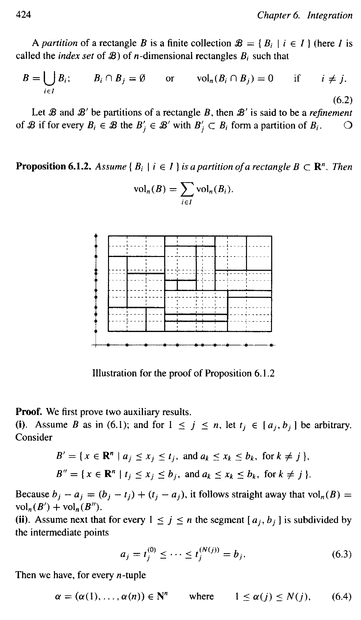Hope that helps ...

Peter

B' is the intersection of those two rectangles. But a partition is a set of rectangles whose union gives you your full set. So you don't have a partition of B'. "Made up of" is not a technical term, and I think it's why you are getting confused.

Oh ... indeed ... can see we should be dealing with intersections ... because in definition of B there are only "ands" ... as we can see:

$B' = \{ ( x_1, x_2 ) \in \mathbb{R^2} \; \; | \; \; a_1 \leq x_1 \leq t_1 \text{ and } a_2 \leq x_2 \leq b_2 \; \; ; \; \; \text{ and } a_2 \leq x_2 \leq t_2 \text{ and } a_1 \leq x_1 \leq b_1 \}$

$B'$ is the intersection of what I have called $B'_1 \text{ and } B'_2$

... and so it follows that

$B"$ is the intersection of what I have called $B"_1 \text{ and } B"_2$

But then from inspecting Figures 1 to 4 one can see that

$\text{vol}_n (B) \neq \text{vol}_n (B') + \text{vol}_n (B'')$ ... ... ... ... (**)

Still reflecting on showing that

$\text{vol}_n (B) = \text{vol}_n (B') + \text{vol}_n (B'')$ ... ... ... ... (*)

By the way, you mention that:

" ... But a partition is a set of rectangles whose union gives you your full set. So you don't have a partition of B' ... ..."

Are you implying that $B' \text{ and } B"$ are partitions of B? or should be ...

Because Duistermaat and Kolk define $B' \text{ and } B"$ without mentioning partitions ...

Peter

Last edited:
B' and B'' do not form a partition, and they do not match what is defined in the stuff in your original post. ##B_1'## and ##B_1''## do form a partition, and do match the ##B'## and ##B''# of the book. You added the other rectangles and took the intersection on your own, changing the object.

•Math Amateur
B' is the intersection of those two rectangles. But a partition is a set of rectangles whose union gives you your full set. So you don't have a partition of B'. "Made up of" is not a technical term, and I think it's why you are getting confused.

Oh ... indeed ... can see we should be dealing with intersections ... because in definition of B there are only "ands" ... as we can see:

$B' = \{ ( x_1, x_2 ) \in \mathbb{R^2} \; \; | \; \; a_1 \leq x_1 \leq t_1 \text{ and } a_2 \leq x_2 \leq b_2 \; \; ; \; \; \text{ and } a_2 \leq x_2 \leq t_2 \text{ and } a_1 \leq x_1 \leq b_1 \}$

$B'$ is the intersection of what I have called $B'_1 \text{ and } B'_2$

... and so it follows that

$B"$ is the intersection of what I have called $B"_1 \text{ and } B"_2$

But then from inspecting Figures 1 to 4 one can see that

$\text{vol}_n (B) \neq \text{vol}_n (B') + \text{vol}_n (B'')$ ... ... ... ... (**)

Peter

B' and B'' do not form a partition, and they do not match what is defined in the stuff in your original post. ##B_1'## and ##B_1''## do form a partition, and do match the ##B'## and ##B''# of the book. You added the other rectangles and took the intersection on your own, changing the object.
You write:

" ... $B' \text{ and } B''$ do not form a partition, and they do not match what is defined in the stuff in your original post. ... "

This is important ... where did i go wrong then in describing $B' \text{ and } B''$? Can you be explicit and help clarify ...?

You write:

" ... $B_1' \text{ and } B_1''$ do form a partition, and do match the $B' \text{ and } B''$ of the book. ... "

This goes to the heart of what i don't understand ... can you explain explicitly why this is the case ... and where i made mistakes ...

I am particularly confused as to how and why $B_1' \text{ and } B_1''$ do match the $B' \text{ and } B''$ of the book. ...

Hope you can help,

Peter

I will now try to simplify and focus my original post ... and try to increase the clarity of my questions ...

At the start of the proof in (i) D&K write:

" ... ... ... for $1 \leq j \leq n, \text{ let } t_j \in [a_j, b_j]$ be arbitrary.

Consider

$$B' = \{ x \in \mathbb{R^n} \ \ | \ \ a_j \leq x_j \leq t_j \text{ and } a_k \leq x_k \leq b_k \text{ for } k \neq j \}$$ ... ... ... "

... now ... to simplify the situation ... consider the case for $n = 2$ ... that is, for $\mathbb{R^2}$

Thus we consider :

$B' = \{ ( x_1, x_2 ) \in \mathbb{R^2} \ \ | \ \ a_j \leq x_j \leq t_j \text{ and } a_k \leq x_k \leq b_k \text{ for } k \neq j \}$

where $1 \leq j \leq 2$

*** Now my problem is how do we validly and correctly determine B' ... ***

The sets involved in determining B' are as follows:

For $j=1$ we consider the set : $a_1 \leq x_1 \leq t_1 \text{ and } a_2 \leq x_2 \leq b_2$

For $j=2$ we consider the set : $a_2 \leq x_2 \leq t_2 \text{ and } a_1 \leq x_1 \leq b_1$

Now ... with these sets ... do we :

$\bullet$ Take $j=1$ or take $j=2$ and just consider one set

$\bullet$ Consider the case for $j=1$ or $j=2$ ... that is , take the union of both sets

$\bullet$ Consider the case for $j=1$ and $j=2$ ... that is , take the intersection of both sets

I think the correct option is to take the intersection of both sets since in the initial specification of B' both conditions seem to apply ...

Is that correct?

If it is not correct can someone please explain the mistakes, shortcomings and deficiencies ...

If the intersection is the valid and correct way to proceed then :

$B' = \{ ( x_1, x_2 ) \in \mathbb{R^2} \ \ | \ \ a_1 \leq x_1 \leq t_1 \text{ and } a_2 \leq x_2 \leq t_2 \}$

Is that correct?

Hoping someone can help .

... any help will be much appreciated ...

Peter

Last edited:
I think I now know what was causing confusion for me in this proof ...

When Duistermaat and Kolk wrote:

" ... ... and for $i \leq j \leq n$, let $t_j \in [a_j, b_j]$ be arbitrary.

Consider

$B' = \{ ( x_1, x_2 ) \in \mathbb{R^2} \; \; | \; \; a_j \leq x_j \leq t_j \text{ and } a_k \leq x_k \leq b_k \text{ for } k \neq i \}$

$B" = \{ ( x_1, x_2 ) \in \mathbb{R^2} \; \; | \; \; t_j \leq x_j \leq b_j \text{ and } a_k \leq x_k \leq b_k \text{ for } k \neq i \}$

... ... ... ... ... "

I thought that one had to let j run through the numbers 1 to n as an index ...

...BUT ...

... now I think that one fixes an arbitrary j and uses this j through B' and B" ...and of course this yields a different analysis ...

! So my troubles were due to interpreting "be arbitrary" wrongly ...

Peter

Last edited:
•Office_Shredder